# Motion in a Straight Line Class 11 NCERT solutions

Question 3.1
In which of the following examples of motion, can the body be considered approximately a point object:
(a) a railway carriage moving without jerks between two stations.
(b) a monkey sitting on top of a man cycling smoothly on a circular track.
(c) a spinning cricket ball that turns sharply on hitting the ground.
(d) a tumbling beaker that has slipped off the edge of a table.
Solution
An object can be treated as point object if the size of the object is much smaller than the distance it moves in a reasonable duration of time.
(a) The size of a carriage is very small as compared to the distance between two stations. Therefore, the carriage can be treated as a point sized object.
(b) The size of a monkey is very small as compared to the size of a circular track. Therefore, the monkey can be considered as a point sized object on the track.
(c) The size of a spinning cricket ball is comparable to the distance through which it turns sharply on hitting the ground. Hence, the cricket ball cannot be considered as a point object.
(d) The size of a beaker is comparable to the height of the table from which it slipped. Hence, the beaker cannot be considered as a point object.
Hence (a) and (b) are correct Solutions
Question 3.2
The position-time (x-t) graphs for two children A and B returning from their school O to their homes P and Q respectively are shown. Choose the correct entries in the brackets below;
(a) (A/B) lives closer to the school than (B/A)
(b) (A/B) starts from the school earlier than (B/A)
(c) (A/B) walks faster than (B/A)
(d) A and B reach home at the (same/different) time
(e) (A/B) overtakes (B/A) on the road (once/twice).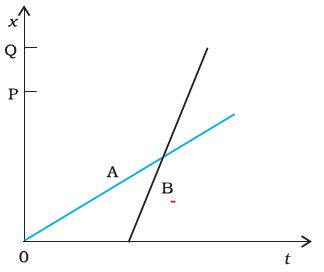Solution
(a) As OP < OQA lives closer to the school than B.
(b) For x = 0, t = 0 for A; while t has some finite value for B. Therefore, A starts from the school earlier than B.
(c) We know that velocity is equal to slope of x-t graph. Now since slope of x-t graph for B is greater than that for A, Therefore B walks faster than A.
(d) It is clear from the given graph that both A and B reach their respective homes at the same time.
(e) The x-t graph intersects only once for A and B. Also, B moves later than A and his/her speed is greater than that of A. Hence B overtakes A only once on the road.

Question 3.3
A woman starts from her home at 9.00 am, walks with a speed of 5 km/h on a straight road up to her office 2.5 km away, stays at the office up to 5.00 pm, and returns home by an auto with a speed of 25 km/hr. Choose suitable scales and plot the x-t graph of her motion.
Solution
Journey to Office
Speed of the woman = 5 km/h
Distance between her office and home = 2.5 km
Time taken = Distance / Speed
= 2.5 / 5 = 0.5 h = 30 min
So, Women starts at 9:00 AM and reaches office by 9:30 AM
Hence between 9:30 AM and 5:00 PM, she stays in the office which is at distance 2.5 Km from home
Journey Back to Home from Office
It is given that she covers the same distance in the evening by an auto.
Now, speed of the auto = 25 km/h
Time taken = Distance / Speed
= 2.5 / 25 = 1 / 10 = 0.1 h = 6 min
Hence, she starts at 5:00 PM from office and reaches home by 5:06 PM
The suitable x-t graph of the motion of the woman is shown in the given figure.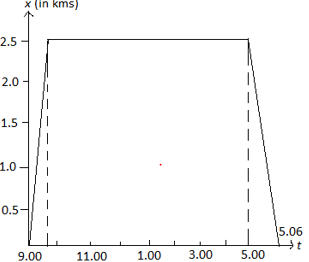Question 3.4
A drunkard walking in a narrow lane takes 5 steps forward and 3 steps backward, followed again by 5 steps forward and 3 steps backward, and so on. Each step is 1 m long and requires 1 s. Plot the x-t graph of his motion. Determine graphically and otherwise how long the drunkard takes to fall in a pit 13 m away from the start.
Solution
We can see from the question
Distance covered with 1 step = 1 m
Time taken = 1 s
Time taken to move first 5 m forward = 5 s
Time taken to move 3 m backward = 3 s
So net distance after 8 secs= 2m
Similarly, we can find the data point for values also
The position of the drunkard taking starting point as position with respect to time is given the below table
 T(s) 0 5 8 13 16 21 24 29 32 37 X(m) 0 5 2 7 4 9 6 11 8 13
The x-t graph based on the above data points is given by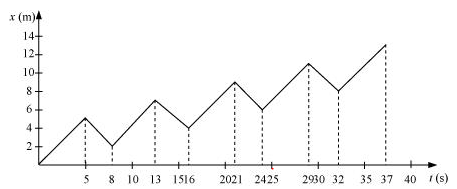So, we can see that, drunkard will fall in pit at 37 sec
Analytical method
Net distance covered after 8sec = 5 – 3 = 2 m
Net time taken to cover 2 m = 8 s
So Drunkard covered 8 m in = 4X8= 32 s.
In the next 5 s, the drunkard will cover a distance of 5 m and a total distance of 13 m and falls into the pit.
Net time taken by the drunkard to cover 13 m = 32 + 5 = 37 s
Question 3.5
A jet airplane travelling at the speed of 500 km/h ejects its products of combustion at the speed of 1500 km/h relative to the jet plane. What is the speed of the latter with respect to an observer on the ground?
Solution
Speed of the jet airplane, v1 = 500 km/h
Let v2 be the speed of product of combustion with respect to ground =v2
Let’s take jet direction as positive direction, then
Relative speed of its products of combustion with respect to the plane,
v21 = – 1500 km/h
v2 -v1   =-1500
v2 =-1500+500=-1000 km/h

The negative sign indicates that the direction of its products of combustion is opposite to the direction of motion of the jet airplane.
Question 3.6
A car moving along a straight highway with speed of 126 km/h is brought to a stop within a distance of 200 m. What is the retardation of the car (assumed uniform), and how long does it take for the car to stop?
Solution
Initial velocity of the car, u = 126 km/h = 35 m/s
Final velocity of the car, v = 0
Distance covered by the car before coming to rest, s = 200 m
Retardation produced in the car = a
From third equation of motion, a can be calculated as:
v2 - u2 = 2as
(0)2 - (35)2 = 2 × a × 200
a = - 35 × 35 / 2 × 200 = - 3.06 m/s2
From first equation of motion, time (t) taken by the car to stop can be obtained as:
v = u + at
t = (v - u) / a = (- 35) / (-3.06) = 11.44 s
Question 3.7
Two trains A and B of length 400 m each are moving on two parallel tracks with a uniform speed of 72 km/h in the same direction, with A ahead of B. The driver of B decides to overtake A and accelerates by 1 m/s2. If after 50 s, the guard of B just brushes past the driver of A, what was the original distance between them?
Solution
For train A:
Initial velocity, u = 72 km/h = 20 m/s
Time, t = 50 s
Acceleration, a = 0 (Since it is moving with a uniform velocity)
From second equation of motion, distance S1 covered by train A can be obtained as:
S1 = ut + (1/2) at2
= 20 × 50 + 0 = 1000 m
For train B:
Initial velocity, u = 72 km/h = 20 m/s
Acceleration, a = 1 m/s2
Time, t = 50 s
From second equation of motion, distance S2 covered by train A can be obtained as:
S2= ut + (1/2) at2
= 20 X 50 + (1/2) × 1 × (50)2 = 2250 m
Hence, the original distance between the driver of train A and the guard of train B is 2250 - 1000  = 1250m.
Question 3.8
On a two-lane road, car A is travelling with a speed of 36 km/h. Two cars B and C approach car A in opposite directions with a speed of 54 km/h each. At a certain instant, when the distance AB is equal to AC, both being 1 km, B decides to overtake A before C does. What minimum acceleration of car B is required to avoid an accident?

Solution
Velocity of car A, vA = 36 km/h = 10 m/s
Velocity of car B, vB = 54 km/h = 15 m/s
Velocity of car C, vC = 54 km/h = 15 m/s
Situation is depicted below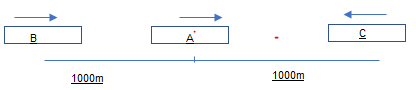Taking direction of Car A as positive
Hence Relative velocity of car B with respect to car A,
vBA = vB – vA
= 15 – 10 = 5 m/s
Relative velocity of car C with respect to car A,
vCA = vvA
= -15 - 10 = -25 m/s
At a certain instance, both cars B and C are at the same distance from car A i.e.,
s = 1 km = 1000 m
Time taken (t) by car C to cover 1000 m = 1000 / 25 = 40 s
Hence, to avoid an accident, car B must cover the same distance in a maximum of 40 s.
From second equation of motion, minimum acceleration (a) produced by car B can be obtained as:
s = ut + (1/2)at2
1000 = 5 × 40 + (1/2) × a × (40)2
a = 1600 / 1600 = 1 m/s

Question 3.9
Two towns A and B are connected by a regular bus service with a bus leaving in either direction every T minutes. A man cycling with a speed of 20 km h-1 in the direction A to B notices that a bus goes past him every 18 min in the direction of his motion, and every 6 min in the opposite direction. What is the period T of the bus service and with what speed (assumed constant) do the buses ply on the road?

Solution
Let V be the speed of the bus running between towns A and B.
Speed of the cyclist, v = 20 km/h
Buses moving in direction A to B
Buses are leaving A every T min. So, distance between two buses plying in same direction at any time would be V(T/60) Km
At an instance when one bus passes through the cycle, so another bus would be V(T/60) Km behind it.
Now that another bus must pass the cyclist in 18 min (as buses passes through cyclist every 18 min). Now this can be solved using Relative Velocity concept
Relative speed of the  another bus moving in the direction of the cyclist
V – v = (V – 20) km/h
Time taken =18min=18/60 h
Distance= V(T/60)
Distance=Relative speed X time
(V - 20) × 18 / 60 = VT / 60     ---(1)
Buses moving in direction B to A

Again, Buses are leaving B every T min. So, distance between two buses plying in same direction at any time would be V(T/60) Km
At an instance when one bus passes through the cycle, so another bus would be V(T/60) Km behind it.
Now that another bus must pass the cyclist in 6 min (as buses passes through cyclist every 6 min)
Relative speed of the bus moving in the opposite direction of the cyclist
= (V + 20) km/h
Time taken by the bus to go past the cyclist = 6 min = 6 / 60 h
(V + 20) × 6 / 60 = VT / 60    .... (2)

From equations (1) and (2), we get
(V + 20) × 6 / 60 = (V - 20) × 18 / 60
V + 20 = 3V - 60
2V = 80
V = 40 km/h
Substituting the value of V in equation (iv), we get
(40 + 20) × 6 / 60 = 40T / 60
T = 360 / 40 = 9 min
Question 3.10
A player throws a ball upwards with an initial speed of 29.4 m/s
(a) What is the direction of acceleration during the upward motion of the ball ?
(b) What are the velocity and acceleration of the ball at the highest point of its motion ?
(c) Choose the x = 0 m and t = 0 s to be the location and time of the ball at its highest point, vertically downward direction to be the positive direction of x-axis, and give the signs of position, velocity and acceleration of the ball during its upward, and downward motion.
(d) To what height does the ball rise and after how long does the ball return to the player’s hands ? (Take g = 9.8 m s-2 and neglect air resistance).

Solution

(a)Irrespective of the direction of the motion of the ball, acceleration (which is acceleration due to gravity) always acts in the downward direction towards the centre of the Earth.
(b)At maximum height, velocity of the ball becomes zero. Acceleration due to gravity at a given place is constant and acts on the ball at all points (including the highest point) with a constant value i.e., 9.8 m/s2.
(c) During upward motion, the sign of position is positive, sign of velocity is negative, and sign of acceleration is positive. During downward motion, the signs of position, velocity, and acceleration are all positive.
(d)Initial velocity of the ball, u = -29.4 m/s
Final velocity of the ball, v = 0 (At maximum height, the velocity of the ball becomes zero)
Acceleration, a = g = 9.8 m/s2
From third equation of motion, height (s) can be calculated as:
v- u2 = 2g(x-x0)
Now x=0
x0 = (v2 - u2/ 2g
= ((0)2 - (-29.4)2/ 2 × (9.8) = 44.1 m

Let t be the time taken to reach the highest point
From First equation of motion
v=u + at
0=-29.4+ 9.8t
t= 3sec
As Time of ascent = Time of descent
Hence, the total time taken by the ball to return to the boy hands = 3 + 3 = 6 s.
Question 3.11
Read each statement below carefully and state with reasons and examples, if it is true or false; A particle in one-dimensional motion
(a) with zero speed at an instant may have non-zero acceleration at that instant
(b) with zero speed may have non-zero velocity,
(c) with constant speed must have zero acceleration,
(d) with positive value of acceleration must be speeding up.
Solution
(a) True, when an object is thrown vertically up in the air, its speed becomes zero at maximum height. However, it has acceleration equal to the acceleration due to gravity (g) that acts in the downward direction at that point.
(b) False, Speed is the magnitude of velocity. When speed is zero, the magnitude of velocity along with the velocity is zero.
(c) True, A car moving on a straight highway with constant speed will have constant velocity. Since acceleration is defined as the rate of change of velocity, acceleration of the car is also zero.
(d) False, If the initial velocity of the body is negative and acceleration is positive Then, for all the time before velocity becomes zero, there is slowing down of the particle. Such a case happens when a particle is projected upwards.
Question 3.12
A ball is dropped from a height of 90 m on a floor. At each collision with the floor, the ball loses one tenth of its speed. Plot the speed-time graph of its motion between t = 0 to 12 s.
Solution
i) Downward motion of Ball
Ball is dropped from a height, s = 90 m
Initial velocity of the ball, = 0
Acceleration, = g = 9.8 m/s2
Final velocity of the ball = v
From second equation of motion, time (t) taken by the ball to hit the ground can be obtained as:
s = ut + (1/2)at2
90 = 0 + (1/2) × 9.8 t2
t = √18.38  = 4.29 s
From first equation of motion, final velocity is given as:
v = u at
= 0 + 9.8 × 4.29 = 42.04 m/s
Here we see that v=at, so velocity varies linearly with downward motion of ball.
This part of motion is represented by first line (1)  in the below graph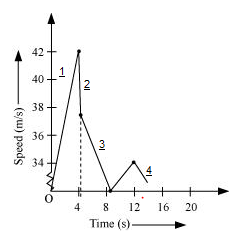ii) First rebound
Now Rebound velocity of the ball, ur =v(1- 1/10) = 9v / 10 = 9 × 42.04 / 10 = 37.84 m/s
This is represented the line (2) in the graph. We are assumed negligible time of collision between ball and floor

iii)  Upward motion of ball
Time (t1) taken by the ball to reach maximum height is obtained with the help of first equation of motion as:
v = u + at1
0 = 37.84 + (– 9.8) t1
t1 = -37.84 / -9.8 = 3.86 s
Total time taken by the ball = t + t1 = 4.29 + 3.86 = 8.15 s
This is represented by line (3) in the graph
iv) Second descent of ball
As the time of ascent is equal to the time of descent, the ball takes 3.86 s to strike back on the floor for the second time.
The velocity with which the ball rebounds from the floor = 9 × 37.84 / 10 = 34.05 m/s
Total time taken by the ball for second rebound = 8.15 + 3.86 = 12.01 s
This is represented by line (4) in the graph
Question 3.13
Explain clearly, with examples, the distinction between:
(a) magnitude of displacement (sometimes called distance) over an interval of time, and the total length of path covered by a particle over the same interval;
(b) magnitude of average velocity over an interval of time, and the Average Speed over the same interval. [Average speed of a particle over an interval of time is defined as the total path length divided by the time interval]. Show in both (a) and (b) that the second quantity is either greater than or equal to the first.
When is the equality sign true? [For simplicity, consider one-dimensional motion only].
Solution
(a) The magnitude of displacement over an interval of time is the shortest distance (which is a straight line) between the initial and final positions of the particle.
The total path length of a particle is the actual path length covered by the particle in each interval of time.
This can be understood with the below example
Suppose a particle moves from point A to point B and then, comes back to a point, C taking a total time t, as shown below.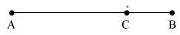Now the magnitude of displacement of the particle is given by = AC.
And total path length is given b = AB + BC
So here Total Path Length > displacement
It is also important to note that the magnitude of displacement can never be greater than the total path length. However, in some cases, both quantities are equal.
Let instead of coming back to C from B, particle moves to position D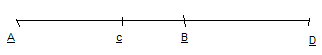Then Magnitude of displacement = AD
Total path length =AB +BD
So, both the quantities are equal now
So, both quantities will be equal if the particle continues to move along a straight line.
(b) Magnitude of average velocity = Magnitude of displacement / Time interval
For the given particle,
Average velocity = AC / t
Average speed = Total path length / Time interval
= (AB + BC) / t
Since (AB + BC) > AC, average speed is greater than the magnitude of average velocity.
Now for the second case
Average speed= (AB +BD)/t
So, both the quantities are equal
So, both quantities will be equal if the particle continues to move along a straight line.
Question 3.14
A man walks on a straight road from his home to a market 2.5 km away with a speed of 5 km/h. Finding the market closed, he instantly turns and walks back home with a speed of 7.5 km/h. What is the
(a) magnitude of average velocity, and
(b) average speed of the man over the interval of time (i) 0 to 30 min, (ii) 0 to 50 min, (iii) 0 to 40 min? [Note: You will appreciate from this exercise why it is better to define average speed as total path length divided by time, and not as magnitude of average velocity. You would not like to tell the tired man on his return home that his average speed was zero!]
Solution
Time taken by the man to reach the market from home, t1 = 2.5/5 = 1/2 h = 30 min
Time taken by the man to reach home from the market, t2 = 2.5/7.5 = 1/3 h = 20 min
Total time taken in the whole journey = 30 + 20 = 50 min
(i) 0 to 30 min
Average velocity = Displacement/Time = 2.5/ (1/2) = 5 km/h
Average speed = Distance/Time = 2.5/ (1/2) = 5 km/h
(ii) 0 to 50 min
Time = 50 min = 50/60 = 5/6 h
Net displacement = 0
Total distance = 2.5 + 2.5 = 5 km
Average velocity = Displacement / Time = 0
Average speed = Distance / Time = 5/ (5/6) = 6 km/h
(iii) 0 to 40 min
Speed of the man = 7.5 km/h
Distance travelled in first 30 min = 2.5 km
Distance travelled by the man (from market to home) in the next 10 min
= 7.5 × 10/60 = 1.25 km
Net displacement = 2.5 – 1.25 = 1.25 km
Total distance travelled = 2.5 + 1.25 = 3.75 km
Average velocity = Displacement / Time = 1.25 / (40/60) = 1.875 km/h
Average speed = Distance / Time = 3.75 / (40/60) = 5.625 km/h
Question 3.15
In Exercises 3.13 and 3.14, we have carefully distinguished between average speed and magnitude of average velocity. No such distinction is necessary when we consider instantaneous speed and magnitude of velocity. The instantaneous speed is always equal to the magnitude of instantaneous velocity. Why?
Solution
Instantaneous velocity is given by the first derivative of distance with respect to time i.e.,
v = dx / dt
Here, the time interval dt is so small that it is assumed that the particle does not change its direction of motion. As a result, both the total path length and magnitude of displacement become equal is this interval of time.
Therefore, instantaneous speed is always equal to instantaneous velocity.
Question 3.16
Look at the graphs (a) to (d) in below figure carefully and state, with reasons, which of these cannot possibly represent one-dimensional motion of a particle.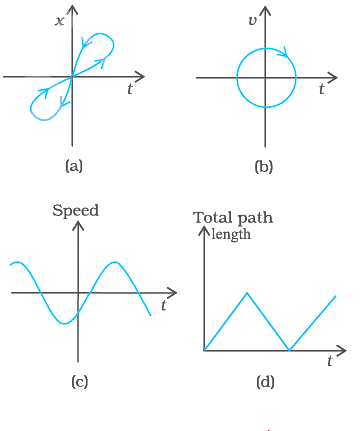Solution
(a) If a vertical line is drawn from any point on axis representing t, we see that it cuts the graph at two points which means particle has two positions at the same instant of time which is not possible. So, the given x-t graph, shown in (a), does not represent one-dimensional motion of the particle
(b)  If a vertical line is drawn from any point on axis representing t, we see that it cuts the graph at two points which means particle has two velocities at the same instant of time which is not possible. So, the given v-t graph, shown in (b), does not represent one-dimensional motion of the particle.
(c) Speed has negative values in the graph which is not possible. So, the given v-t graph, shown in (c), does not represent one-dimensional motion of the particle.
(d) The given v-t graph, shown in (d), does not represent one-dimensional motion of the particle. This is because the total path length travelled by the particle cannot decrease with time.

Question 3.17
Below figure shows the x-t plot of one-dimensional motion of a particle. Is it correct to say from the graph that the particle moves in a straight line for t < 0 and on a parabolic path for t > 0? If not, suggest a suitable physical context for this graph.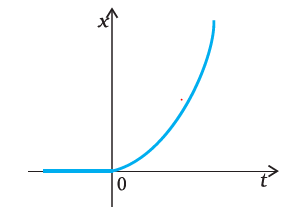Solution
No, because the x-t graph does not represent the trajectory of the path followed by a particle. From the graph, it is noted that at t=0, x=0

Question 3.18
A police van moving on a highway with a speed of 30 km/h fires a bullet at a thief’s car speeding away in the same direction with a speed of 192 km/h. If the muzzle speed of the bullet is 150 m/s, with what speed does the bullet hit the thief’s car? (Note: Obtain that speed which is relevant for damaging the thief’s car).

Solution
Speed of the police van, vp = 30 km/h = 8.33 m/s
Muzzle speed of the bullet, vb = 150 m/s
Speed of the thief’s car, vt= 192 km/h = 53.33 m/s
Since the bullet is fired from a moving van, its resultant speed can be obtained as:
= 150 + 8.33 = 158.33 m/s
Since both the vehicles are moving in the same direction, the velocity with which the bullet hits the thief’s car can be obtained as:
vbt = vb – vt
= 158.33 – 53.33 = 105 m/s
Question 3.19
Suggest a suitable physical situation for each of the following graphs in below figure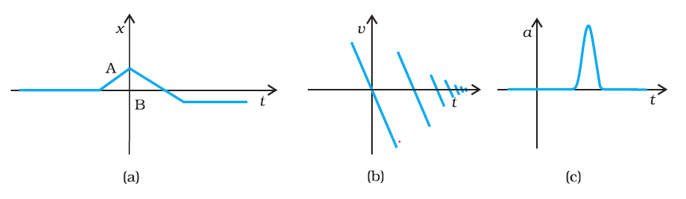Solution
(a) The given x-t graph shows that initially a body was at rest. Then, its velocity increases with time and attains an instantaneous constant value. The velocity then reduces to zero with an increase in time. Then, its velocity increases with time in the opposite direction and acquires a constant value. A similar physical situation arises when a football (initially kept at rest) is kicked and gets rebound from a rigid wall so that its speed gets reduced. Then, it passes from the player who has kicked it and ultimately gets stopped after some time.
(b) In the given v-t graph, the sign of velocity changes and its magnitude decreases with a passage of time. A similar situation arises when a ball is dropped on the hard floor from a height. It strikes the floor with some velocity and upon rebound, its velocity decreases by a factor. This continues till the velocity of the ball eventually becomes zero.
(c) The given a-t graph reveals that initially the body is moving with a certain uniform velocity. Its acceleration increases for a short interval of time, which again drops to zero. This indicates that the body again starts moving with the same constant velocity. A similar physical situation arises when a hammer moving with a uniform velocity strikes a nail.
Question 3.20
Below figure gives the x-t plot of a particle executing one-dimensional simple harmonic motion. (You will learn about this motion in more detail in Chapter14). Give the signs of position, velocity and acceleration variables of the particle at t = 0.3 s, 1.2 s, - 1.2 s.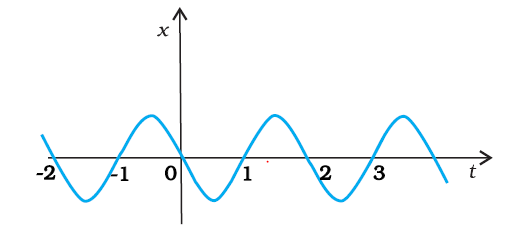Solution
For simple harmonic motion (SHM) of a particle, acceleration (a) is given by the relation:
a = – ω2x
Where ω = angular frequency
t = 0.3 s
In this time interval, x is negative. Now the slope of the x-t plot is negative. Therefore, both position and velocity are negative. Acceleration of the particle using (i) will be positive.
t = 1.2 s
In this time interval, x is positive. Now the slope of the x-t plot is positive. Therefore, both position and velocity are positive. Acceleration of the particle using (i) will be negative.
t = – 1.2 s
In this time interval, x is negative. Now the slope of the x-t plot is positive. Therefore, position is negative and velocity is positive. Acceleration of the particle using (i) will be positive.

Question 3.21
Below figure gives the x-t plot of a particle in one-dimensional motion. Three different equal intervals of time are shown. In which interval is the average speed greatest, and in which is it the least? Give the sign of average velocity for each interval.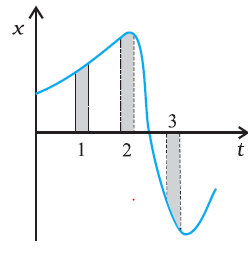Solution
The average speed of a particle shown in the x-t graph is obtained from the slope of the graph in an interval of time.
It is clear from the graph that the slope is maximum and minimum restively in intervals 3 and 2 respectively. Therefore, the average speed of the particle is the greatest in interval 3 and is the least in interval 2. The sign of average velocity is positive in both intervals 1 and 2 as the slope is positive in these intervals. However, it is negative in interval 3 because the slope is negative in this interval.

Question 3.22
Figure 3.25 gives a speed-time graph of a particle in motion along a constant direction. Three equal intervals of time are shown. In which interval is the average acceleration greatest in magnitude? In which interval is the average speed greatest? Choosing the positive direction as the constant direction of motion, give the signs of v and a in the three intervals. What are the accelerations at the points A, B, C and D?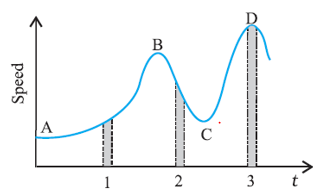Solution
i) As the change of speed is greatest in interval 2, So magnitude of acceleration is greatest in interval 2
ii) Height of the curve from the time-axis gives the average speed of the particle. It is apparent from the graph that height is the greatest in interval 3. Hence, average speed of the particle is the greatest in interval 3.
iii) v is positive in all the intervals
Acceleration is positive in interval 1 and 3 as speed is increasing while it is negative in interval 2 as speed is decreasing
iv) Points A, B, C, and D are all parallel to the time-axis. Hence, the slope is zero at these points. Therefore, at points A, B, C, and D, acceleration of the particle is zero.

Question 3.23
A three-wheeler starts from rest, accelerates uniformly with 1 m/s2 on a straight road for 10 s, and then moves with uniform velocity. Plot the distance covered by the vehicle during the nth second (n = 1,2,3….) versus n. What do you expect this plot to be during accelerated motion: a straight line or a parabola?

Solution
Distance covered by a body in nth second is given by the relation
Sn = u + a (2n - 1)/2     .... (i)
Where,
u = Initial velocity
a = Acceleration
= Time = 1, 2, 3, .... , n
In the given case,
u = 0 and a = 1 m/s2
∴ Sn = (2n - 1) / 2     .... (ii)
Which is a linear equation, so graph would be a straight line
Now, substituting different values of n in equation (ii), we get the following table:
 n 1 2 3 4 5 6 7 8 9 10 Sn 0.5 1.5 2.5 3.5 4.5 5.5 6.5 7.5 8.5 9.5

The plot between and S will be a straight line shown in below figure: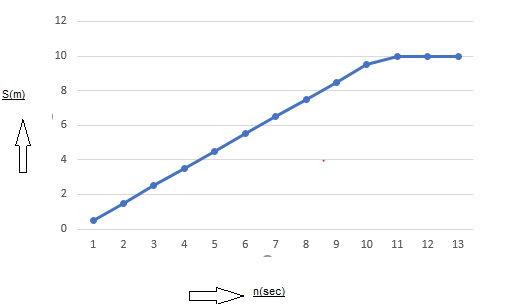After 10 secs, speed of the three-wheeler has become
V=10X1=10 m/s
So, it will cover 10 m in each sec which is represented by the horizontal line in the graph

Question 3.24
A boy standing on a stationary lift (open from above) throws a ball upwards with the maximum initial speed he can, equal to 49 m s-1. How much time does the ball take to return to his hands? If the lift starts moving up with a uniform speed of 5 m s-1 and the boy again throws the ball up with the maximum speed he can, how long does the ball take to return to his hands?

Solution
Initial velocity of the ball, u = 49 m/s
Acceleration, a = – g = – 9.8 m/s2

Case I:
When the lift was stationary, the boy throws the ball.
Taking upward motion of the ball,
Final velocity, v of the ball becomes zero at the highest point.
From first equation of motion, time of ascent (t) is given as:
v = u +at
t = (v - u/ a
= -49 / -9.8 = 5 s
But, the time of ascent is equal to the time of descent.
Hence, the total time taken by the ball to return to the boy’s hand = 5 + 5 = 10 s.
Case II:
The lift was moving up with a uniform velocity of 5 m/s. In this case, the relative velocity of the ball with respect to the boy remains the same i.e., 49 m/s. Therefore, in this case also, the ball will return back to the boy’s hand after 10 s.
Question 3.25
On a long horizontally moving belt (below figure), a child runs to and fro with a speed 9 km /h (with respect to the belt) between his father and mother located 50 m apart on the moving belt. The belt moves with a speed of 4 km h-1. For an observer on a stationary platform outside, what is the
(a) speed of the child running in the direction of motion of the belt ?.
(b) speed of the child running opposite to the direction of motion of the belt?
(c) time taken by the child in (a) and (b)?
Which of the Solutions alter if motion is viewed by one of the parents?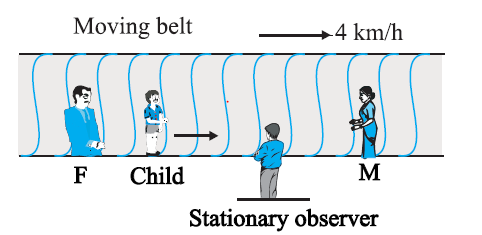Solution
(a)Speed of the belt, v1 = 4 km/h
Speed of the boy w.r.t belt, v2 = 9 km/h
Since the boy is running in the same direction of the motion of the belt, his speed (as observed by the stationary observer) can be obtained as:
v = v1 + v2 = 4 + 9 = 13 km/h
(b)Since the boy is running in the direction opposite to the direction of the motion of the belt, his speed (as observed by the stationary observer) can be obtained as:
v = v1 + (– v2) = 4 – 9 = -5 km/h (negative as child is moving in opposite direction)
(c) Distance between the child’s parents = 50 m
As both parents are standing on the moving belt, the speed of the child in either direction as observed by the parents will remain the same i.e., 9 km/h = 2.5 m/s.
Hence, the time taken by the child to move towards one of his parents is given by
= 50 / 2.5 = 20 s
(d)If the motion is viewed by any one of the parents, Solutions obtained in (a) and (b) get altered. This is because the child and his parents are standing on the same belt and hence, are equally affected by the motion of the belt. Therefore, for both parents (irrespective of the direction of motion) the speed of the child remains the same i.e., 9 km/h.
For this reason, it can be concluded that the time taken by the child to reach any one of his parents remains unaltered.
Question 3.26
Two stones are thrown up simultaneously from the edge of a cliff 200 m high with initial speeds of 15 m/s and 30 m/s. Verify that the graph shown in below figure correctly represents the time variation of the relative position of the second stone with respect to the first. Neglect air resistance and assume that the stones do not rebound after hitting the ground. Take g = 10 m s-2. Give the equations for the linear and curved parts of the plot.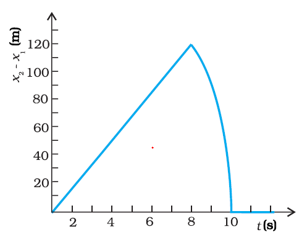Solution
For first stone:
Initial velocity, u = 15 m/s
Acceleration, a = –g = – 10 m/s2
Using the relation,
x1 = x0 + u1t + (1/2)at2
Where, height of the cliff, x0 = 200 m
x1 = 200 + 15t - 5t2      ......(i)
When this stone hits the ground, x1 = 0
– 5t+ 15t + 200 = 0
t2 – 3t – 40 = 0
t2 – 8t + 5t – 40 = 0
(t – 8) + 5 (t – 8) = 0
t = 8 s or = – 5 s
Since the stone was projected at time t = 0, the negative sign before time is meaningless.
t = 8 s
For second stone:
Initial velocity, u = 30 m/s
Acceleration, a = – g = – 10 m/s2
Using the relation,
x2 = x0 + ut + (1/2)at2
= 200 + 30t - 5t2........(ii)
At the moment when this stone hits the ground; x2 = 0
– 5t2 + 30 t + 200 = 0
t2 – 6t – 40 = 0
t2 – 10t + 4t + 40 = 0
t (t – 10) + 4 (t – 10) = 0
t (t – 10) (t + 4) = 0
t = 10 s or t = – 4 s
Here again, the negative sign is meaningless.
∴ t = 10 s
Subtracting equations (i) and (ii), we get
x2 - x1 = (200 + 30t -5t2) - (200 + 15t -5t2)
x2 - x1 =15t     ....... (iii)
Equation (iii) represents the linear path of both stones. Due to this linear relation between (x– x1) and t, the path remains a straight line till 8 s.
Maximum separation between the two stones is at t = 8 s.
(x2 – x1)max = 15× 8 = 120 m
This is in accordance with the given graph.
After 8 s, only second stone is in motion whose variation with time is given by the Quadratic equation:
x2 – x= 200 + 30t – 5t2
Hence, the equation of linear and curved path is given by
x– x1 = 15t (Linear path)
x2 ­– x1 = 200 + 30t – 5t2 (Curved path)
After 10 secs, both the stone are on the ground, so relative distance is zero and this is represented by horizontal line after 10 secs
Question 3.27
The speed-time graph of a particle moving along a fixed direction is shown in below figure. Obtain the distance traversed by the particle between
(a) t = 0 s to 10 s
(b) t = 2 s to 6 s.
(c)What is the average speed of the particle over the intervals in (a) and (b)?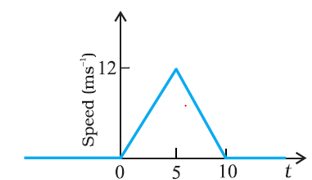Solution
(a) Distance travelled by the particle = Area under the given graph
= (1/2) × (10 - 0) × (12 - 0) = 60 m
Average speed = Distance / Time = 60 / 10 = 6 m/s
(b)Let s1 and s2 be the distances covered by the particle between time
= 2 s to 5 s and t = 5 s to 6 s respectively.
Total distance (s) covered by the particle in time t = 2 s to 6 s
s = s1 + s2
For distance s1
Let u1 be the velocity of the particle after 2 s and a1 be the acceleration of the particle in t = 0 to t = 5 s.
Since the particle undergoes uniform acceleration in the interval t = 0 to t = 5 s, from first equation of motion, acceleration can be obtained as:
at
Where,
v = Final velocity of the particle
12 = 0 +a1 × 5
a1= 12 / 5 = 2.4 m/s2
Again, from first equation of motion, we have velocity at t=2sec
at
u1= 0 + 2.4 × 2 = 4.8 m/s
Distance travelled by the particle between time 2 s and 5 s i.e., in 3 s
s1 = u1 t + (1/2)a1 t2
= 4.8 × 3 + (1/2) × 2.4 × (3)2
= 25.2 m      ........(i)
For distance s2:
Let a2 be the acceleration of the particle between time t = 5 s and t = 10 s.
From first equation of motion,
at (where v = 0 as the particle finally comes to rest)
0 = 12 + a2 × 5
a2 = -12 / 5 = - 2.4 m/s2
Distance travelled by the particle in 1s (i.e., between = 5 s and t = 6 s)
s2 = u2 t + (1/2)a2 t2
= 12 × 1 + (1/2) (-2.4) × (1)2
= 12 - 1.2 = 10.8 m    .........(ii)
From equations (i) and (ii) we get
s = 25.2 + 10.8 = 36 m
Therefore,
Average speed = Total distance/time taken = 36 / 4 = 9 m/s
Question 3.28
The velocity-time graph of a particle in one-dimensional motion is shown in below figure :
Which of the following formulae are correct for describing the motion of the particle over the time-interval t1 to t2 :
(a) x(t2 ) = x(t1) + v (t1) (t2 – t1) +(½) a (t2 – t1)2
(b) v(t2 ) = v(t1) + a (t2 – t1)
(c) vverage = (x(t2) – x(t1))/(t2 – t1)
(d) aaverage = (v(t2) – v(t1))/(t2 – t1)
(e) x(t2 ) = x(t1) + vaverage (t2 – t1) + (½) aaverage (t2 – t1)2
(f) x(t2 ) – x(t1) = area under the v-t curve bounded by the t-axis and the dotted line shown.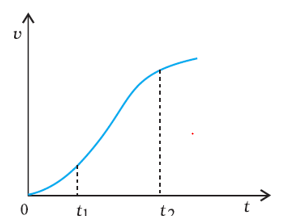Solution
The correct formulae describing the motion of the particle are (c), (d) and, (f)
The given graph has a non-uniform slope. Hence, the formulae given in (a), (b), and (e) cannot describe the motion of the particle. Only relations given in (c), (d), and (f) are correct equations of motion.